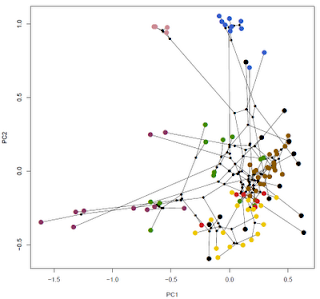## Saturday, February 26, 2011

### Ecomorph phylomorphospaceCheck out the neat plot created by Luke Mahler using my phylomorphospace() function (available here). Click on the image at right to enlarge. In this plot are the phenotypes and phylogenetic relationships among 100 Greater Antillean Anolis lizards. According to Luke, PC1 is limb lengths (more or less); and PC2 is overall size. The tips of the tree are color coded by ecomorph. The phylomorphospace graphing method is based on Sidlauskas (2008).

Luke made a few modifications/additions to my code to obtain this plot. First, he filled and shrunk the points that are the inferred states at internal nodes. He did this by changing the following line of code:

points(x=c(X[tree\$edge[i,1],1],X[tree\$edge[i,2],1]),
y=c(X[tree\$edge[i,1],2],X[tree\$edge[i,2],2]),
col=c(con\$col.node[as.character(tree\$edge[i,1]),1],
con\$col.node[as.character(tree\$edge[i,2]),1]))

to this:

points(x=c(X[tree\$edge[i,1],1],X[tree\$edge[i,2],1]),
y=c(X[tree\$edge[i,1],2],X[tree\$edge[i,2],2]),
col=c(con\$col.node[as.character(tree\$edge[i,1]),1],
con\$col.node[as.character(tree\$edge[i,2]),1]),pch=16,cex=0.8)

To plot the large, color coded tip nodes, he merely created the vector color.code.e, containing the species color coded by ecomorph; and then plotted his raw data for PCs 1 & 2 on top of his phylomorphospace graph as follows:

> points(X[,1],X[,2],col=(color.code.e),pch=16,cex=1.7)

Thanks to Luke for sharing this.

#### 1 comment:

1.Note: due to the very large amount of spam, all comments are now automatically submitted for moderation.